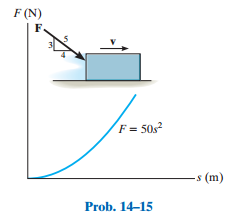### Create an Account

Home / Questions / The force F acting in a constant direction on the 20-kg block has a magnitude which varie...

# The force F acting in a constant direction on the 20-kg block has a magnitude which varies with position s of the block Determine the speed of the block after it slides 3 m When the block is movi

The force F, acting in a constant direction on the 20-kg block, has a magnitude which varies with position s of the block. Determine the speed of the block after it slides 3 m. When the block is moving to the right at The coefficient of kinetic friction between the block and surface is mk = 0.3. s = 0 2 m>s. mk = 0.3. 2 m/s. The coefficient of kinetic friction between the block and surface is  µk = 0.3.Jun 03 2020 View more View LessSubscribe To Get Solution